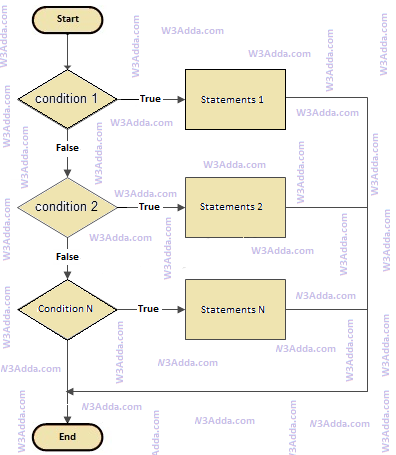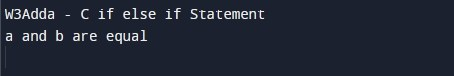# C if else if Statement

In this tutorial you will learn about the C if else if Statement and its application with practical example.

## C if else if Statement

In C programming, the if-else if statement allows us to add an alternative set of test conditions in the if..else statement using else-if and single else statements for the if condition. In such way if..else.if statement is used to select one among several blocks of code to be executed.

### C if..else..if Statement Flow Diagramc-if-else-if-statement

### if else if Statement Syntax

Below is the general syntax of the if-else if statement in c programming:

Syntax:-

### if-else if Statement Example

Below is a simple example to demonstrate the use of if-else if statement in c programming:

Example:-

Output:-In this tutorial we have learn about the C if else if Statement and its application with practical example. I hope you will like this tutorial.